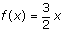Definition Of Independent Variable

Independent variable is a variable in an equation, whose values make up the domain.
In other words, an independent variable in an equation may have its value freely chosen regardless the values of any other variable.

Example of Independent Variable

In the equation y = 7x + 5, the independent variable is x. The variable y is not independent, because it depends on the value chosen for x

Solved Example on Independent Variable

Ques: What is the independent variable in the function?Choices:

A. f(x)
B. 3/2
C. x
D. no independent variable